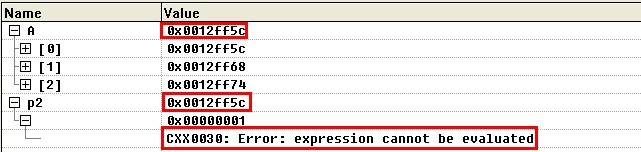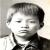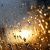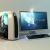## C++中二位数组的指针到底该如何使用？

```int A={{1,2,3},{4,5,6},{7,8,9}};

int *p1=A;
int **p2=(int**)A;
int t1=A;
int t2=p1;
int t3=**A;
int t4=*(*(A+1)+1);
int t5=(*(A+1));
int t6=**p2;
int t7=*(*(p2+1)+1);
int t8=(*(p2+1));```

`int t6=**p2;`

`int t7=*(*(p2+1)+1);`

`int t8=(*(p2+1));`### 以下是问题补充：

@剑神：我换到Ubuntu下的Eclipse也是直接奔溃；换到Ubuntu的Anjuta编译虽然可以过，但是却不能对 t6、t7、t8进行使用 ，一旦使用也会直接奔溃。 (2013/11/30 23:42)

0“p2A的所指地址的值一模一样” ，这个话是你自己说的，哈，可不是编译器和c语言标准说的。你自己理解错误。

int **p2，我说过无数次，是一个空间，名字叫做p2，里面存的数据，用于指向其他空间，不过对其他空间的类型有要求。要求是，后者空间里面存放的仍然是个地址，那个地址将指向int类型的空间。

int a[][]，是二维数组而已。a实际对应的地址并没有任何地方存储，仅表示一个连续的空间，可以被二维网格化。a直接等同于这个空间的首地址。

a和a，你转成整数是，如果他们数值上是否一样，你自己试试就知道。a,a,&(a)他们是否一样你也可以试试就知道。

```int *p1;
int **p2 = p1;
int a;
p1 =&(a);
p1 =&(a);
p1 =&(a);
```0
m

00#### 引用来自“阿汞”的答案0Thank You All!